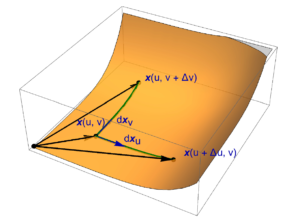## New version of Geometric Algebra for Electrical Engineers posted.A new version of Geometric Algebra for Electrical Engineers (V0.1.8) is now posted.  This fixes a number of issues in Chapter II on geometric calculus.  In particular, I had confused definitions of line, area, and volume integrals that were really the application of the fundamental theorem to such integrals.  This is now fixed, and the whole chapter is generally improved and clarified.

## Generalizing Ampere’s law using geometric algebra.

The question I’d like to explore in this post is how Ampere’s law, the relationship between the line integral of the magnetic field to current (i.e. the enclosed current)
\begin{equation}\label{eqn:flux:20}
\oint_{\partial A} d\Bx \cdot \BH = -\int_A \ncap \cdot \BJ,
\end{equation}
generalizes to geometric algebra where Maxwell’s equations for a statics configuration (all time derivatives zero) is
\begin{equation}\label{eqn:flux:40}
\end{equation}
where the multivector fields and currents are
\begin{equation}\label{eqn:flux:60}
\begin{aligned}
F &= \BE + I \eta \BH \\
J &= \eta \lr{ c \rho – \BJ } + I \lr{ c \rho_\txtm – \BM }.
\end{aligned}
\end{equation}
Here (fictitious) the magnetic charge and current densities that can be useful in antenna theory have been included in the multivector current for generality.

My presumption is that it should be possible to utilize the fundamental theorem of geometric calculus for expressing the integral over an oriented surface to its boundary, but applied directly to Maxwell’s equation. That integral theorem has the form
\begin{equation}\label{eqn:flux:80}
\int_A d^2 \Bx \boldpartial F = \oint_{\partial A} d\Bx F,
\end{equation}
where $$d^2 \Bx = d\Ba \wedge d\Bb$$ is a two parameter bivector valued surface, and $$\boldpartial$$ is vector derivative, the projection of the gradient onto the tangent space. I won’t try to explain all of geometric calculus here, and refer the interested reader to , which is an excellent reference on geometric calculus and integration theory.

The gotcha is that we actually want a surface integral with $$\spacegrad F$$. We can split the gradient into the vector derivative a normal component
\begin{equation}\label{eqn:flux:160}
\end{equation}
so
\begin{equation}\label{eqn:flux:100}
=
\int_A d^2 \Bx \boldpartial F
+
\int_A d^2 \Bx \ncap \lr{ \ncap \cdot \spacegrad } F,
\end{equation}
so
\begin{equation}\label{eqn:flux:120}
\begin{aligned}
\oint_{\partial A} d\Bx F
&=
\int_A d^2 \Bx \lr{ J – \ncap \lr{ \ncap \cdot \spacegrad } F } \\
&=
\int_A dA \lr{ I \ncap J – \lr{ \ncap \cdot \spacegrad } I F }
\end{aligned}
\end{equation}

This is not nearly as nice as the magnetic flux relationship which was nicely split with the current and fields nicely separated. The $$d\Bx F$$ product has all possible grades, as does the $$d^2 \Bx J$$ product (in general). Observe however, that the normal term on the right has only grades 1,2, so we can split our line integral relations into pairs with and without grade 1,2 components
\begin{equation}\label{eqn:flux:140}
\begin{aligned}
&=
\int_A dA \gpgrade{ I \ncap J }{0,3} \\
&=
\int_A dA \lr{ \gpgrade{ I \ncap J }{1,2} – \lr{ \ncap \cdot \spacegrad } I F }.
\end{aligned}
\end{equation}

Let’s expand these explicitly in terms of the component fields and densities to check against the conventional relationships, and see if things look right. The line integrand expands to
\begin{equation}\label{eqn:flux:180}
\begin{aligned}
d\Bx F
&=
d\Bx \lr{ \BE + I \eta \BH }
=
d\Bx \cdot \BE + I \eta d\Bx \cdot \BH
+
d\Bx \wedge \BE + I \eta d\Bx \wedge \BH \\
&=
d\Bx \cdot \BE
– \eta (d\Bx \cross \BH)
+ I (d\Bx \cross \BE )
+ I \eta (d\Bx \cdot \BH),
\end{aligned}
\end{equation}
the current integrand expands to
\begin{equation}\label{eqn:flux:200}
\begin{aligned}
I \ncap J
&=
I \ncap
\lr{
\frac{\rho}{\epsilon} – \eta \BJ + I \lr{ c \rho_\txtm – \BM }
} \\
&=
\ncap I \frac{\rho}{\epsilon} – \eta \ncap I \BJ – \ncap c \rho_\txtm + \ncap \BM \\
&=
\ncap \cdot \BM
+ \eta (\ncap \cross \BJ)
– \ncap c \rho_\txtm
+ I (\ncap \cross \BM)
+ \ncap I \frac{\rho}{\epsilon}
– \eta I (\ncap \cdot \BJ).
\end{aligned}
\end{equation}

We are left with
\begin{equation}\label{eqn:flux:220}
\begin{aligned}
\oint_{\partial A}
\lr{
d\Bx \cdot \BE + I \eta (d\Bx \cdot \BH)
}
&=
\int_A dA
\lr{
\ncap \cdot \BM – \eta I (\ncap \cdot \BJ)
} \\
\oint_{\partial A}
\lr{
– \eta (d\Bx \cross \BH)
+ I (d\Bx \cross \BE )
}
&=
\int_A dA
\lr{
\eta (\ncap \cross \BJ)
– \ncap c \rho_\txtm
+ I (\ncap \cross \BM)
+ \ncap I \frac{\rho}{\epsilon}
-\PD{n}{} \lr{ I \BE – \eta \BH }
}.
\end{aligned}
\end{equation}
This is a crazy mess of dots, crosses, fields and sources. We can split it into one equation for each grade, which will probably look a little more regular. That is
\begin{equation}\label{eqn:flux:240}
\begin{aligned}
\oint_{\partial A} d\Bx \cdot \BE &= \int_A dA \ncap \cdot \BM \\
\oint_{\partial A} d\Bx \cross \BH
&=
\int_A dA
\lr{
– \ncap \cross \BJ
+ \frac{ \ncap \rho_\txtm }{\mu}
– \PD{n}{\BH}
} \\
\oint_{\partial A} d\Bx \cross \BE &=
\int_A dA
\lr{
\ncap \cross \BM
+ \frac{\ncap \rho}{\epsilon}
– \PD{n}{\BE}
} \\
\oint_{\partial A} d\Bx \cdot \BH &= -\int_A dA \ncap \cdot \BJ \\
\end{aligned}
\end{equation}
The first and last equations could have been obtained much more easily from Maxwell’s equations in their conventional form more easily. The two cross product equations with the normal derivatives are not familiar to me, even without the fictitious magnetic sources. It is somewhat remarkable that so much can be packed into one multivector equation:
\begin{equation}\label{eqn:flux:260}
\oint_{\partial A} d\Bx F
=
I \int_A dA \lr{ \ncap J – \PD{n}{F} }.
\end{equation}

# References

 A. Macdonald. Vector and Geometric Calculus. CreateSpace Independent Publishing Platform, 2012.